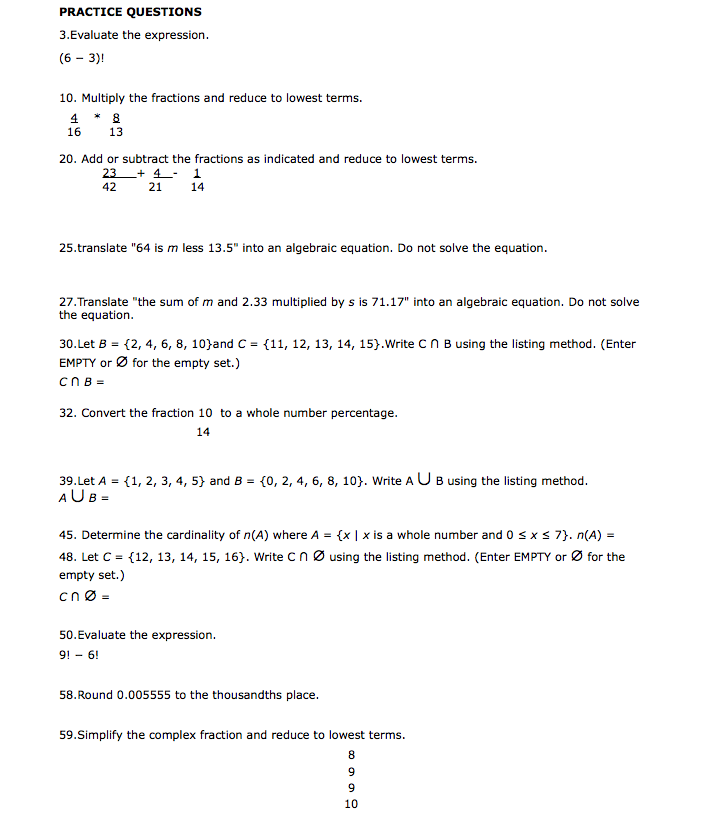# PRACTICE QUESTIONS 3.Evaluate the expression. (6 - 3)! 10. Multiply the fractions and reduce to lowest terms. 16 13 20. Add or subtract the fractions as indicated and reduce to lowest terms. 23 + 4_- 1 21 42 14 25.translate "64 is m less 13.5" into an algebraic equation. Do not solve the equation. 27. Translate "the sum of m and 2.33 multiplied by s is 71.17" into an algebraic equation. Do not solve the equation. 30. Let B = {2, 4, 6, 8, 10}and C = {11, 12, 13, 14, 15}.Write CN B using the listing method. (Enter EMPTY or Ø for the empty set.) CNB = 32. Convert the fraction 10 to a whole number percentage. 14 39.Let A = {1, 2, 3, 4, 5} and B = {0, 2, 4, 6, 8, 10}. Write A UB using the listing method. AUB = 45. Determine the cardinality of n(A) where A = {x | x is a whole number and 0 sx s 7}. n(A) = 48. Let C = {12, 13, 14, 15, 16}. Write cnø using the listing method. (Enter EMPTY or Ø for the empty set.) CnØ = 50.Evaluate the expression. 9! - 6! 58.Round 0.005555 to the thousandths place. 59.Simplify the complex fraction and reduce to lowest terms. 9. 9. 10

Question

Hi I need help with some practice questions that I am stuck in. Thanks for your help.help_outlineImage TranscriptionclosePRACTICE QUESTIONS 3.Evaluate the expression. (6 - 3)! 10. Multiply the fractions and reduce to lowest terms. 16 13 20. Add or subtract the fractions as indicated and reduce to lowest terms. 23 + 4_- 1 21 42 14 25.translate "64 is m less 13.5" into an algebraic equation. Do not solve the equation. 27. Translate "the sum of m and 2.33 multiplied by s is 71.17" into an algebraic equation. Do not solve the equation. 30. Let B = {2, 4, 6, 8, 10}and C = {11, 12, 13, 14, 15}.Write CN B using the listing method. (Enter EMPTY or Ø for the empty set.) CNB = 32. Convert the fraction 10 to a whole number percentage. 14 39.Let A = {1, 2, 3, 4, 5} and B = {0, 2, 4, 6, 8, 10}. Write A UB using the listing method. AUB = 45. Determine the cardinality of n(A) where A = {x | x is a whole number and 0 sx s 7}. n(A) = 48. Let C = {12, 13, 14, 15, 16}. Write cnø using the listing method. (Enter EMPTY or Ø for the empty set.) CnØ = 50.Evaluate the expression. 9! - 6! 58.Round 0.005555 to the thousandths place. 59.Simplify the complex fraction and reduce to lowest terms. 9. 9. 10 fullscreen